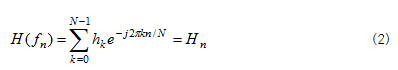When you collect data, you can get data in analog-type waveform with continuous time and amplitude. At first, you have to change such continuous analog signals to discrete digital signals to enable computer to process them. Signals changed into digital type are time series data changing over time. However, when finding information required and analyzing it, it is not sufficient to conduct analysis only at time domain. Transformation of time series signals into frequency domain will enable you to identify frequency components of signals. So, you can conduct various analysis. Then, how such data of time domain can be transformed into those of frequency domain? Fourier Transform is the mathematical processing method for such transformation. Fourier Transform formula can be defined as follow;Collected data, h(t), are those of time domain, which can be transformed into data of frequency domain, H(f), through the above formula. However, since discrete digital signals are at present used, Discrete Fourier Transform (DFT) is used. DFT formula can be defined as follow;This is a process of transforming discrete signals of time domain, hk, into discrete signals of frequency domain, H(fn). This DFT requires very long time to complete calculation owing to its mathematical characteristics. FFT (Fast Fourier Transform) was designed by Danielson and Lanczoc in 1942. In general, DFT requires calculation time equivalent to the square of the number of data (N2), but FFT reduces such time to N log 2N.   In other words, the calculation time, formerly increased by geometric progression in proportion to the number of data, can be reduced by more than a half. FFT, a method for efficient calculation of DFT, has greatly contributed to development of digital signal processing technology.

If signals of time domain are transformed into those of frequency domain by FFT method, the signals are arranged in the graph depending on size of frequency, enabling identification of frequency components of signals.